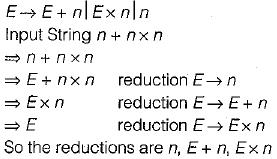Courses

# Test: Syntax Directed Translation- 1

## 8 Questions MCQ Test Question Bank for GATE Computer Science Engineering | Test: Syntax Directed Translation- 1

Description
This mock test of Test: Syntax Directed Translation- 1 for Computer Science Engineering (CSE) helps you for every Computer Science Engineering (CSE) entrance exam. This contains 8 Multiple Choice Questions for Computer Science Engineering (CSE) Test: Syntax Directed Translation- 1 (mcq) to study with solutions a complete question bank. The solved questions answers in this Test: Syntax Directed Translation- 1 quiz give you a good mix of easy questions and tough questions. Computer Science Engineering (CSE) students definitely take this Test: Syntax Directed Translation- 1 exercise for a better result in the exam. You can find other Test: Syntax Directed Translation- 1 extra questions, long questions & short questions for Computer Science Engineering (CSE) on EduRev as well by searching above.
QUESTION: 1

### The term “environment” in programming language semantics is said as

Solution:
QUESTION: 2

Solution:

A linker or link editor is a computer program that takes one or more object files generated by a compiler and combines then into single executable file, library file or another object file.

QUESTION: 3

### Generation of intermediate code based on an abstract machine model is useful in compilers because

Solution:

Generation of intermediate code based on an abstract machine model is useful in compilers because it makes implementation of lexical analysis and syntax analysis easier

QUESTION: 4

In the following grammar: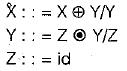Which of the following is true?

Solution:

Creating syntax tree for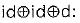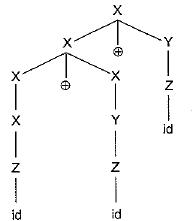Therefore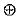is left associative.

Creating syntax tree for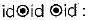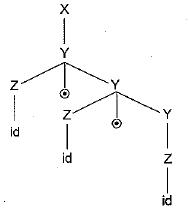Therefore,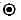is right associative.

QUESTION: 5

In a bottom-up evaluation of a syntax directed definition, inherited attributes can

Solution:

Every S(Synthesized) - attributed definition is L- attributed. For implementing inherited attributed during bottom-up parsing, extends to some, but not LR grammars. Consider the following example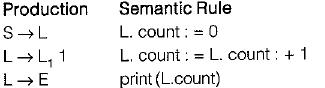In the example above the nonterminal L in L → E inherits the count of the number of 1 ’s generated by S. Since the production L → E is the first that a bottom- up parser would reduce by, the translator at the time can't know the number of 1 ’s in the input. So in a bottom-up evaluation of a syntax directed definition, inherits attributes can’t be evaluated if the definition is L-attributed in the given example. So we can say. that L-attributed definition is based on simple LR(1) grammar, but it can’t be implemented always but inherit attributes can be evaluated only if the definition has synthesized attributes.

QUESTION: 6

Consider the translation scheme shown below: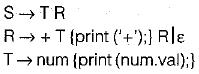Here num is a token that represents an integer and num.val represents the corresponding integer value. For an input string ‘9 + 5 + 2’, this translation scheme will print

Solution:

For the input ‘9 + 5 + 2’ the translation scheme is 95 + 2 + shown below: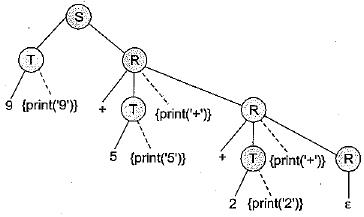QUESTION: 7

Consider the grammar rule E → E1 - E2 for arithmetic expressions. The code generated is targeted to a CPU having a single user register. The subtraction operation requires the first operand to be in the register. If E1 and E2 do not have any common subexpression, in order to get the shortest possible code

Solution:

To optimize the solution evaluate the expression E2. Then we can calculate E1 and finally E1 will be one of operands that will be in register and we can perform subtraction directly. But if we follow the opposite then we have to make move and store operations.

QUESTION: 8

Consider the grammar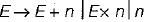For a sentence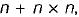, the handles in the right-sentential form of the reduction are

Solution: